# Inequalities Higher GCSE Questions AQA These questions are

• Slides: 27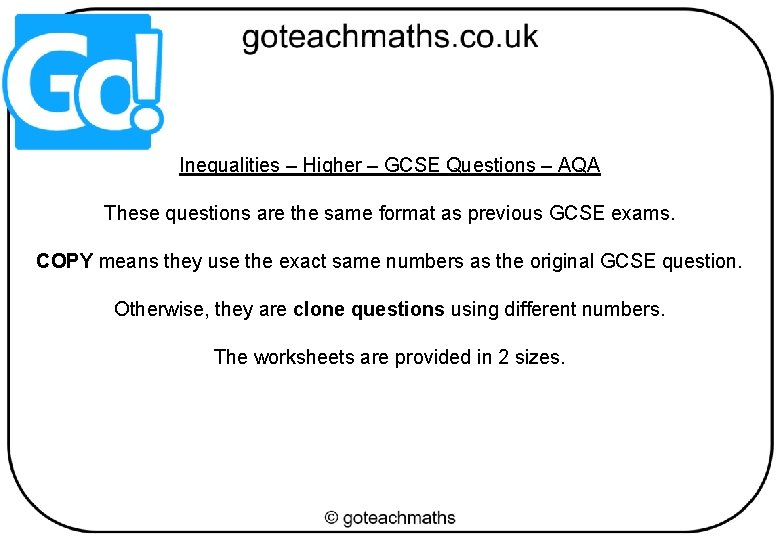Inequalities – Higher – GCSE Questions – AQA These questions are the same format as previous GCSE exams. COPY means they use the exact same numbers as the original GCSE question. Otherwise, they are clone questions using different numbers. The worksheets are provided in 2 sizes.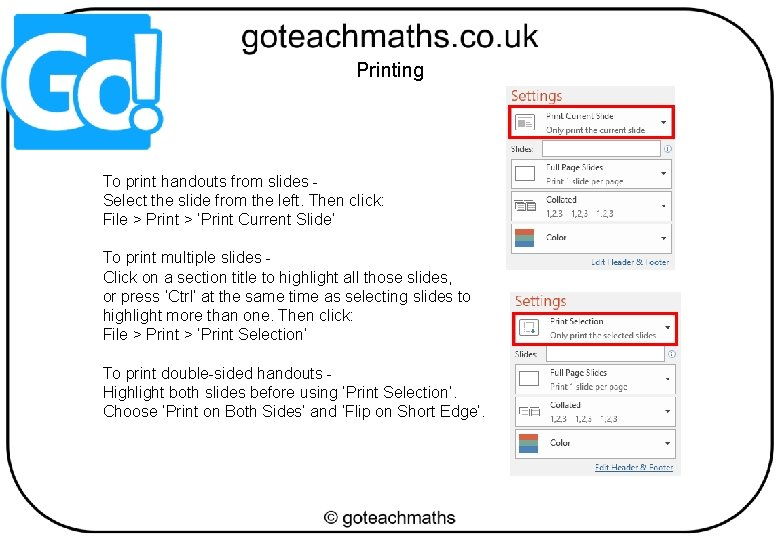Printing To print handouts from slides Select the slide from the left. Then click: File > Print > ‘Print Current Slide’ To print multiple slides Click on a section title to highlight all those slides, or press ‘Ctrl’ at the same time as selecting slides to highlight more than one. Then click: File > Print > ‘Print Selection’ To print double-sided handouts Highlight both slides before using ‘Print Selection’. Choose ‘Print on Both Sides’ and ‘Flip on Short Edge’.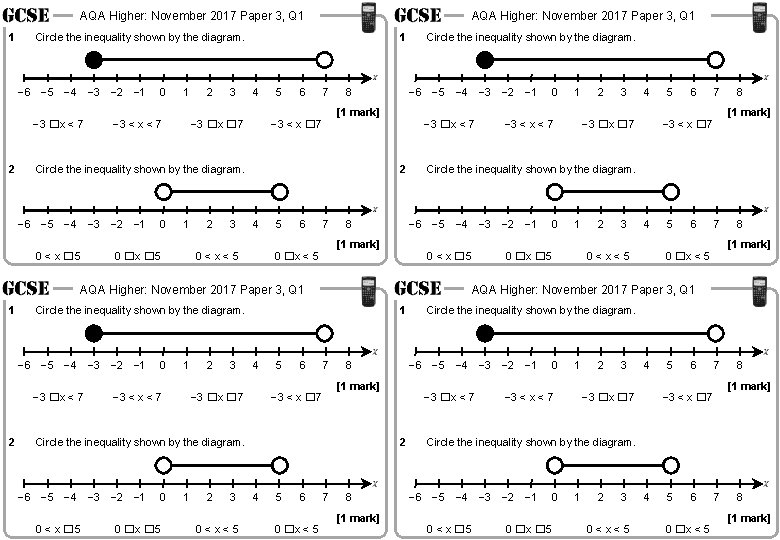AQA Higher: November 2017 Paper 3, Q 1 1 Circle the inequality shown by the diagram. − 6 − 5 − 4 − 3 − 2 − 1 − 3 �x < 7 2 AQA Higher: November 2017 Paper 3, Q 1 0 1 − 3 < x < 7 2 3 1 4 − 3 �x � 7 5 6 7 8 Circle the inequality shown by the diagram. − 6 − 5 − 4 − 3 − 2 − 1 0 < x � 5 0 1 0 �x � 5 2 3 − 6 − 5 − 4 − 3 − 2 − 1 [1 mark] − 3 < x � 7 − 3 �x < 7 2 4 0<x<5 5 6 7 8 1 − 3 �x < 7 2 0 1 − 3 < x < 7 2 3 1 4 − 3 �x � 7 5 6 7 8 0 < x � 5 0 0 �x � 5 1 2 3 0<x<5 5 6 0 �x < 5 4 5 6 7 8 [1 mark] − 3 < x � 7 0 1 2 3 4 0<x<5 5 6 7 8 [1 mark] 0 �x < 5 7 8 [1 mark] Circle the inequality shown by the diagram. − 3 �x < 7 2 4 3 − 3 �x � 7 0 �x � 5 − 6 − 5 − 4 − 3 − 2 − 1 [1 mark] − 3 < x � 7 Circle the inequality shown by the diagram. − 6 − 5 − 4 − 3 − 2 − 1 2 AQA Higher: November 2017 Paper 3, Q 1 Circle the inequality shown by the diagram. − 6 − 5 − 4 − 3 − 2 − 1 1 Circle the inequality shown by the diagram. 0 < x � 5 AQA Higher: November 2017 Paper 3, Q 1 0 − 3 < x < 7 − 6 − 5 − 4 − 3 − 2 − 1 [1 mark] 0 �x < 5 Circle the inequality shown by the diagram. 0 1 − 3 < x < 7 2 3 4 − 3 �x � 7 5 6 7 8 [1 mark] − 3 < x � 7 Circle the inequality shown by the diagram. − 6 − 5 − 4 − 3 − 2 − 1 0 < x � 5 0 0 �x � 5 1 2 3 0<x<5 4 5 6 0 �x < 5 7 8 [1 mark]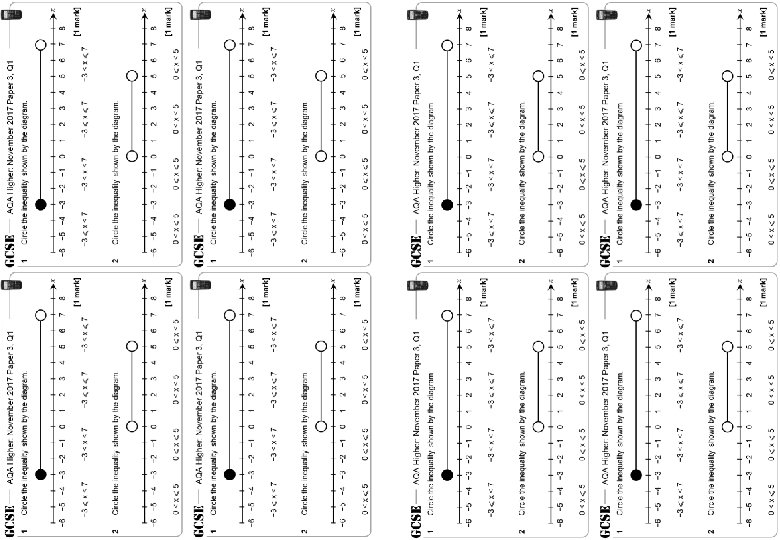AQA Higher: June 2018 Paper 3, Q 2 1 2 AQA Higher: June 2018 Paper 3, Q 2 1 [1 mark] − 3, − 2, − 1, 0, 1, 2 − 2, − 1, 0, 1, 2 − 3, − 2, − 1, 0, 1 2 [1 mark] − 7, − 6, − 5, − 3 − 8, − 7, − 6, − 5, − 4 − 8, − 6, − 5, − 4, − 3 AQA Higher: June 2018 Paper 3, Q 2 1 [1 mark] − 3, − 2, − 1, 0, 1, 2 − 2, − 1, 0, 1, 2 − 3, − 2, − 1, 0, 1 2 [1 mark] − 7, − 6, − 5, − 3 − 8, − 7, − 6, − 5, − 4 − 8, − 6, − 5, − 4, − 3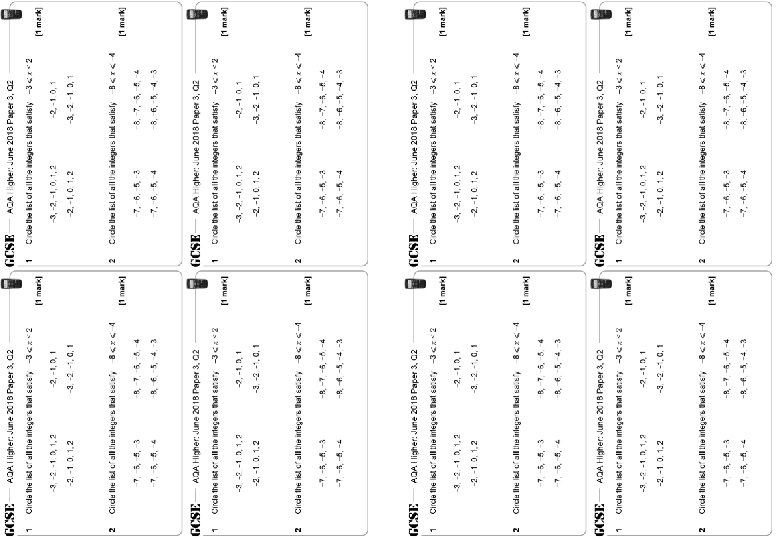AQA Higher: May 2017 Paper 1, Q 14 1 AQA Higher: May 2017 Paper 1, Q 14 [1 mark] 1 Answer 2 Answer [1 mark] 2 Answer 3 [1 mark] 3 AQA Higher: May 2017 Paper 1, Q 14 [1 mark] 1 Answer 2 [1 mark] Answer 3 [1 mark] Answer AQA Higher: May 2017 Paper 1, Q 14 [1 mark] Answer 1 [1 mark] 3 [1 mark] Answer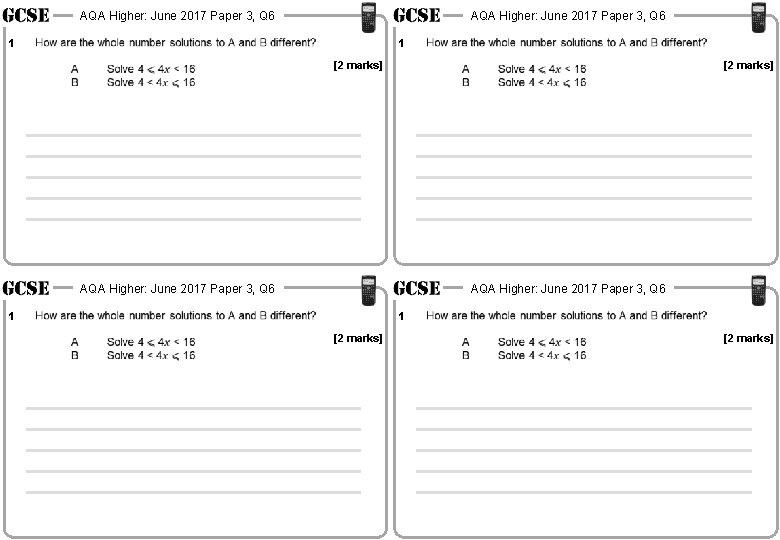AQA Higher: June 2017 Paper 3, Q 6 1 [2 marks] [2 marks]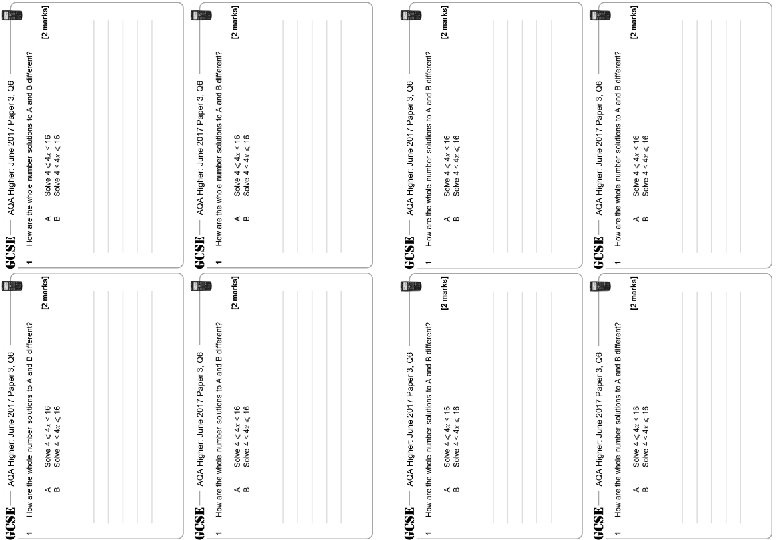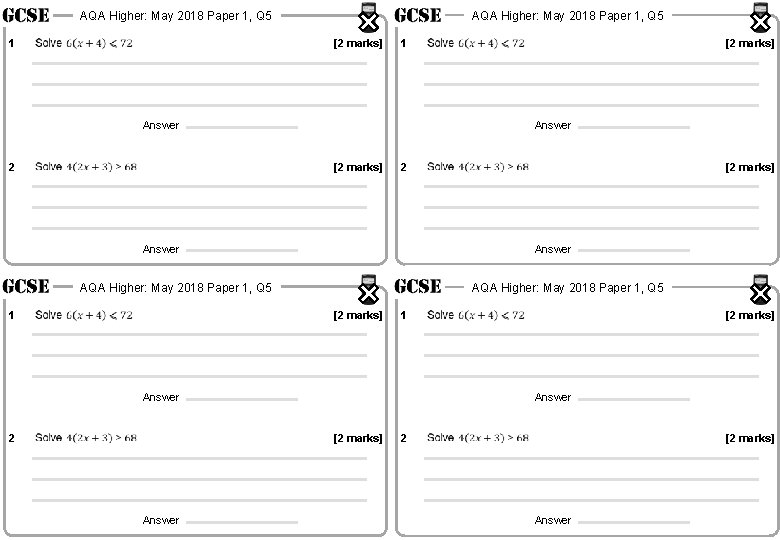AQA Higher: May 2018 Paper 1, Q 5 1 AQA Higher: May 2018 Paper 1, Q 5 [2 marks] 1 Answer 2 Answer [2 marks] 2 Answer AQA Higher: May 2018 Paper 1, Q 5 [2 marks] 1 Answer 2 [2 marks] Answer AQA Higher: May 2018 Paper 1, Q 5 1 [2 marks] 2 [2 marks] Answer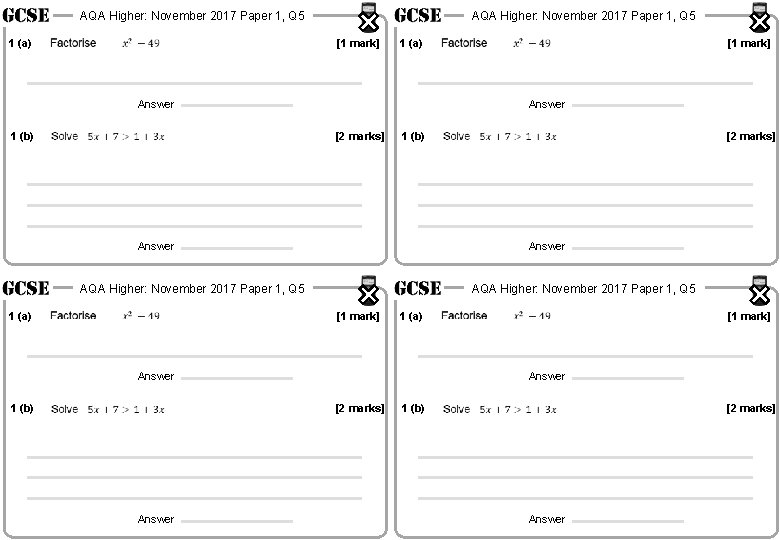AQA Higher: November 2017 Paper 1, Q 5 1 (a) AQA Higher: November 2017 Paper 1, Q 5 [1 mark] 1 (a) Answer 1 (b) Answer [2 marks] 1 (b) Answer AQA Higher: November 2017 Paper 1, Q 5 [1 mark] 1 (a) Answer 1 (b) [1 mark] Answer [2 marks] Answer AQA Higher: November 2017 Paper 1, Q 5 1 (a) [1 mark] 1 (b) [2 marks] Answer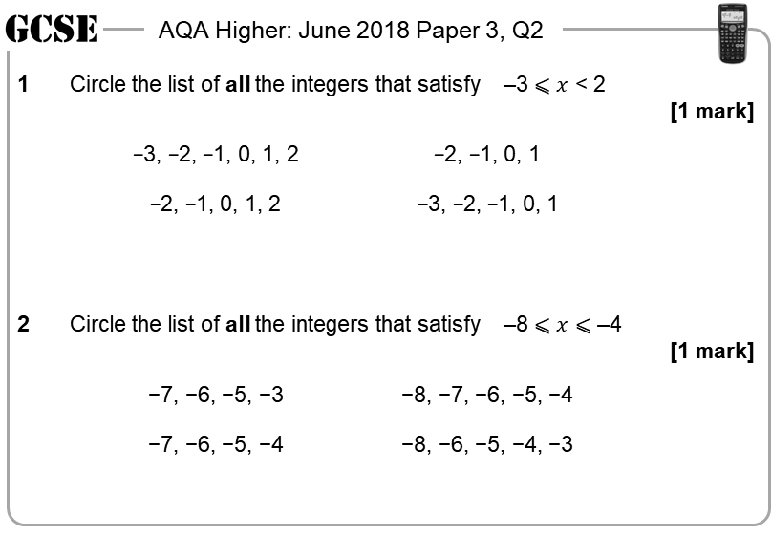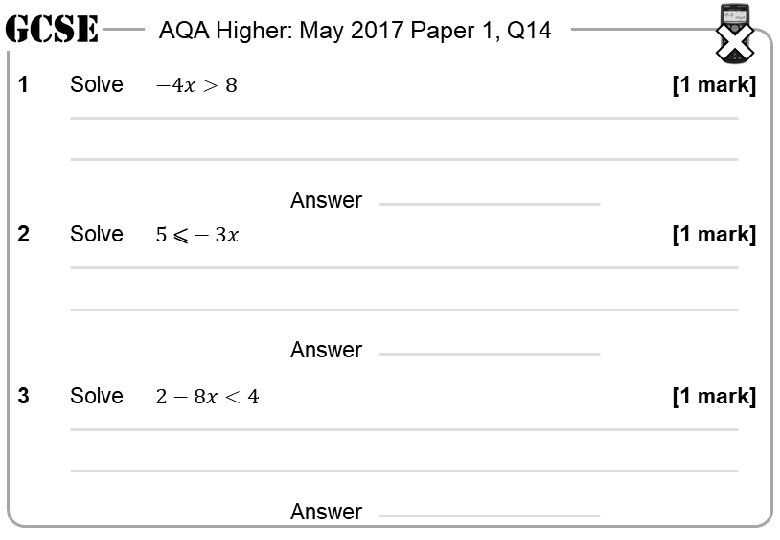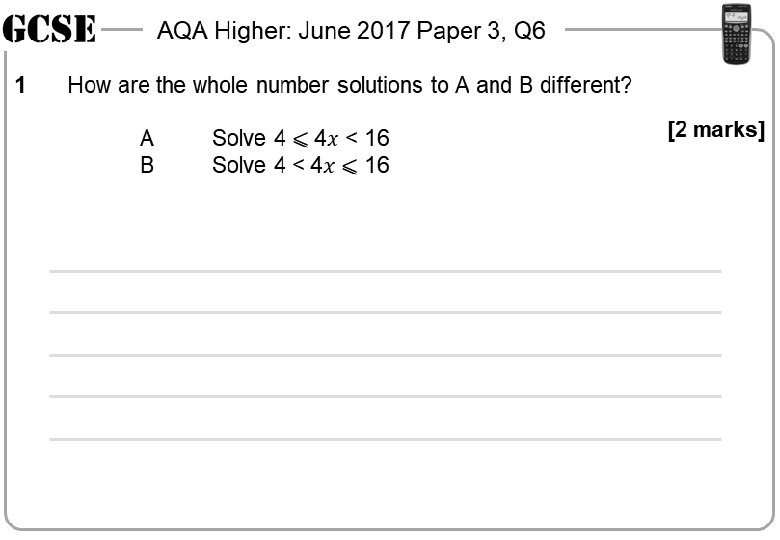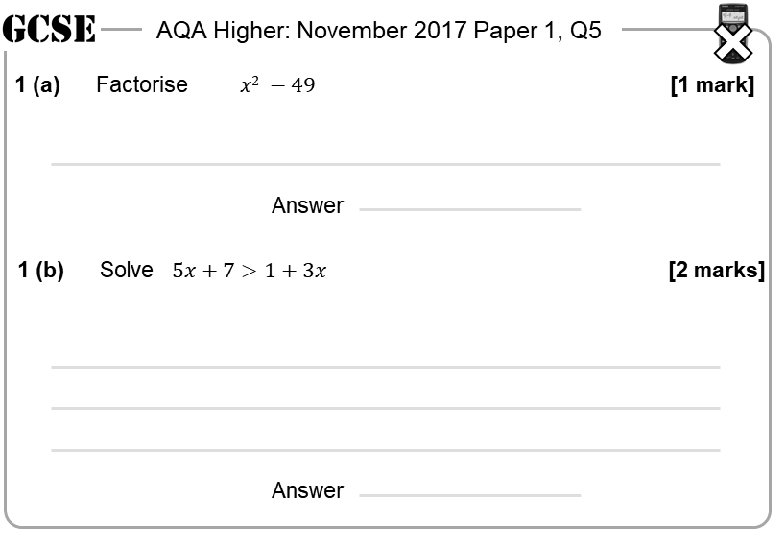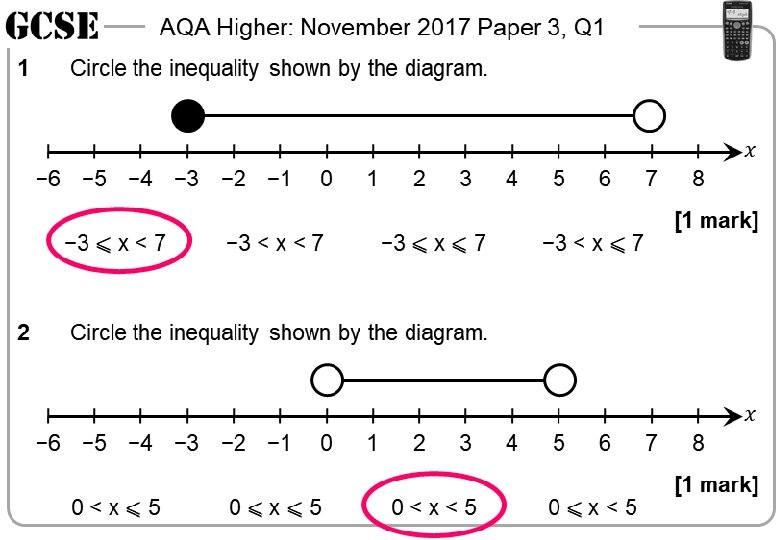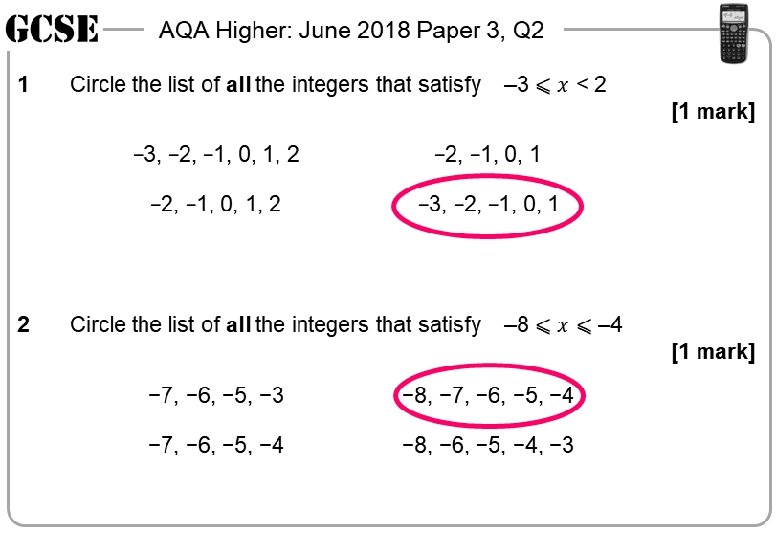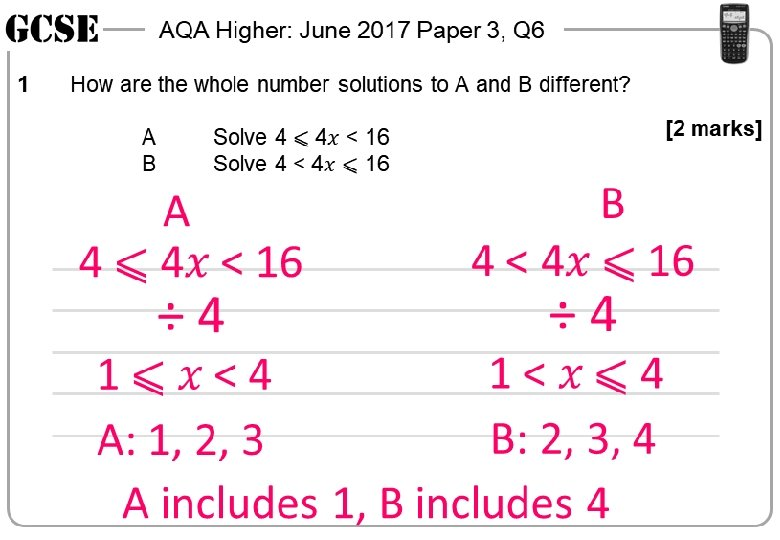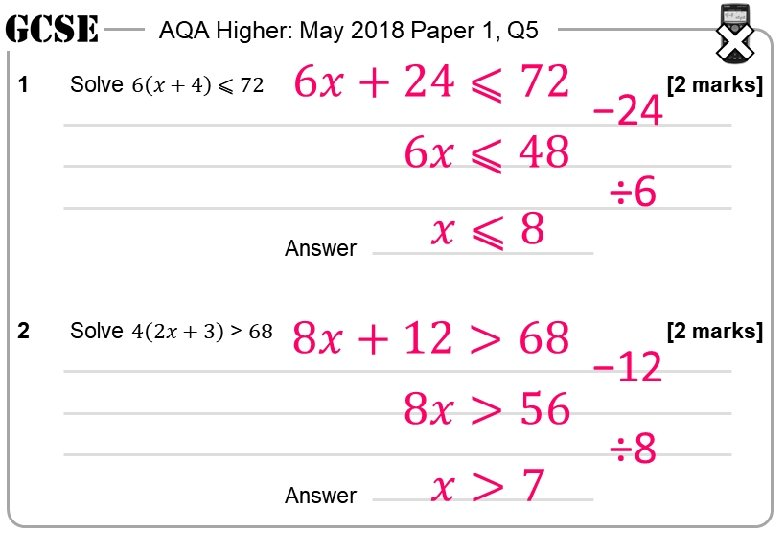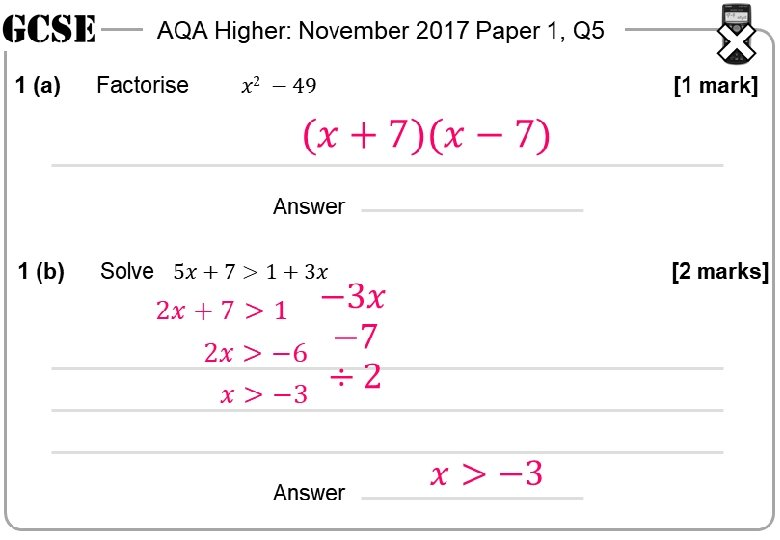Questions? Comments? Suggestions? …or have you found a mistake!? Any feedback would be appreciated . Please feel free to email: [email protected] co. uk